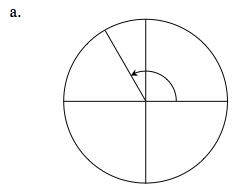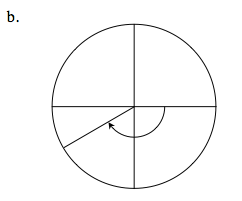### Home > PC > Chapter 2 > Lesson 2.3.7 > Problem2-162

2-162.
1. Below are two of the special angles that are used in the unit circle. Identify the radian measure for the angles shown. Remember counter-clockwise angles are positive and clockwise angles are negative. Homework Help ✎

1.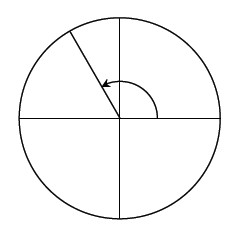2.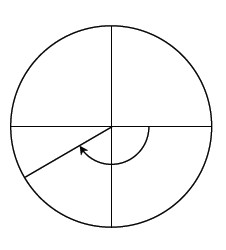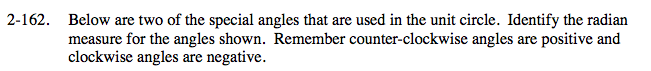$\text{Special angles are at the }\frac{\pi}{6},\frac{\pi}{3},\frac{\pi}{2},\text{ or at the }\frac{\pi}{4}\text{ radians.}$